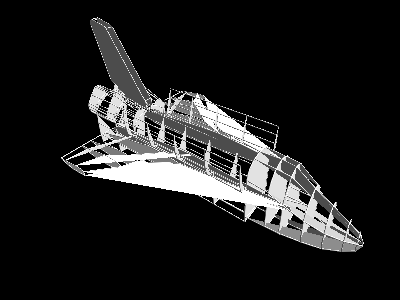# aUCBLogo Demos and Tests / shuttle;   This is a MSWLogo 3D example where I have written a simple ".3DV"
;   file viewer. 3DV is a simple public domain format for 3D Vectors.

;   Do not confuse how this "Shuttle" database was built.
;   The vectors were not built with MSWLogo (although they could be).
;   Do not confuse how you "normally" build 3D objects in MSWLogo.
;   You normally build 3D object just like you always built them
;   in 2D, by moving the turtle around.

;   This example was just done for a completeness test. The data is
;   absolute and uses SETXYZ for all drawing. No turtle.

;   It's a good example of how to use file and Array operations.

;   It's also an example of seeing how I took "foreign" data from the
;   internet and used it in logo. There is TONS of "data" out their.
;   Mars weather statistics, continental drift statistics, etc. that
;   can be used for all sorts of projects. (George Mills)

;   I`ve added the 3DV_display_solid procedure (Andreas Micheler).

be shuttle

local [start finish command vertex]

ht

perspective

cs
;   setEye {400 400 600}{0 0 0}{0 1 0}

command=[]

vertex=[]

pr [ESC stops, + - changes speed]

start=timefine   ; Time and Display Shuttle

setMaterialSpecular HSB 70 0.5 0.5

setMaterialShininess 10

setLightSpotExponent 2

setPenColor 7

setScreenColor 0

;   3DV_display_wireframe command vertex

3DV_display_solid command vertex

finish=timefine-start

show (sentence count command "3D "Commands "in finish "seconds)

; It takes about 90 seconds on a PII 300

rotatescene2
end

openRead file         ; Open Vectors Commands

vertices=first readList      ; Get # of vertices (1st record of file)

vertex=(array vertices 1)      ; Now build a "Vertex" array based on # of vertices

repeat vertices

[            ; Now read in each "Vertex". Format [X Y Z]

]

commands=first readList      ; Get # of "commands" (comes after all vertices)

command=(array commands 1)   ; Now build a "Command" array based on # of commands

repeat commands

[            ; Now read in each "Command". Format [Vertex_Index Color]

]

close file
end

be 3DV_display_wireframe command vertex

local [vtx cmd]

repeat count command

[

cmd=command.repcount ; Get a command.
; Format [VertexIndex Color] Color = 0 means "Move"

vtx=vertex.(first cmd)   ; Get the vertex. Format [X Y Z]

ifelse (last cmd) == 0

[   pu         ; If color 0 then Move

][   pd         ; else Draw (I'm ingoring the color, not RGB Yuck)

]

setPosXYZ vtx         ; Move or draw to the vertex

]
end

be 3DV_display_solid command vertex

local [points cmd commands vertices]

points=[]

commands=count command

vertices=count vertex

penup

enableCylinderLines

setPenSize [2 2]

repeat commands

[   cmd=int command.repcount   ; Get a command.
; Format [VertexIndex Color] Color = 0 means "Move"

vtx=vertex.(first cmd)   ; Get the vertex. Format [X Y Z]

if (last cmd) == 0

[            ; If color = 0 ...

ifelse (count points) == 0

[         ; ..and no points then just move

setPosXYZ vtx

][   if (count points) == 2

[            ; ...and 2 points then connect them

setPosXYZ first points

pd  setPosXYZ last points  pu

]

if (count points) > 2

[            ; ...and 3 or more points then

repeat (count points)-1

[         ; connect them...

setPosXYZ points.repcount

pd  setPosXYZ points.(repcount+1)  pu

]

;setPosXYZ first points
;setPosXYZ last points

pd

while [not empty? points]

[   normal=cross points.1-points.2 points.2-points.3

if (Norm normal) > 1e-1

[   break

]

points=bf points

]

PolyStart         ; and make a polygon out of them.

foreach points

[   setPosXYZ ?

]

PolyEnd

pu

]

]

points=[]      ; finally clear the old points

]

queue "points vtx         ; add the new vertex to the points list

]
end